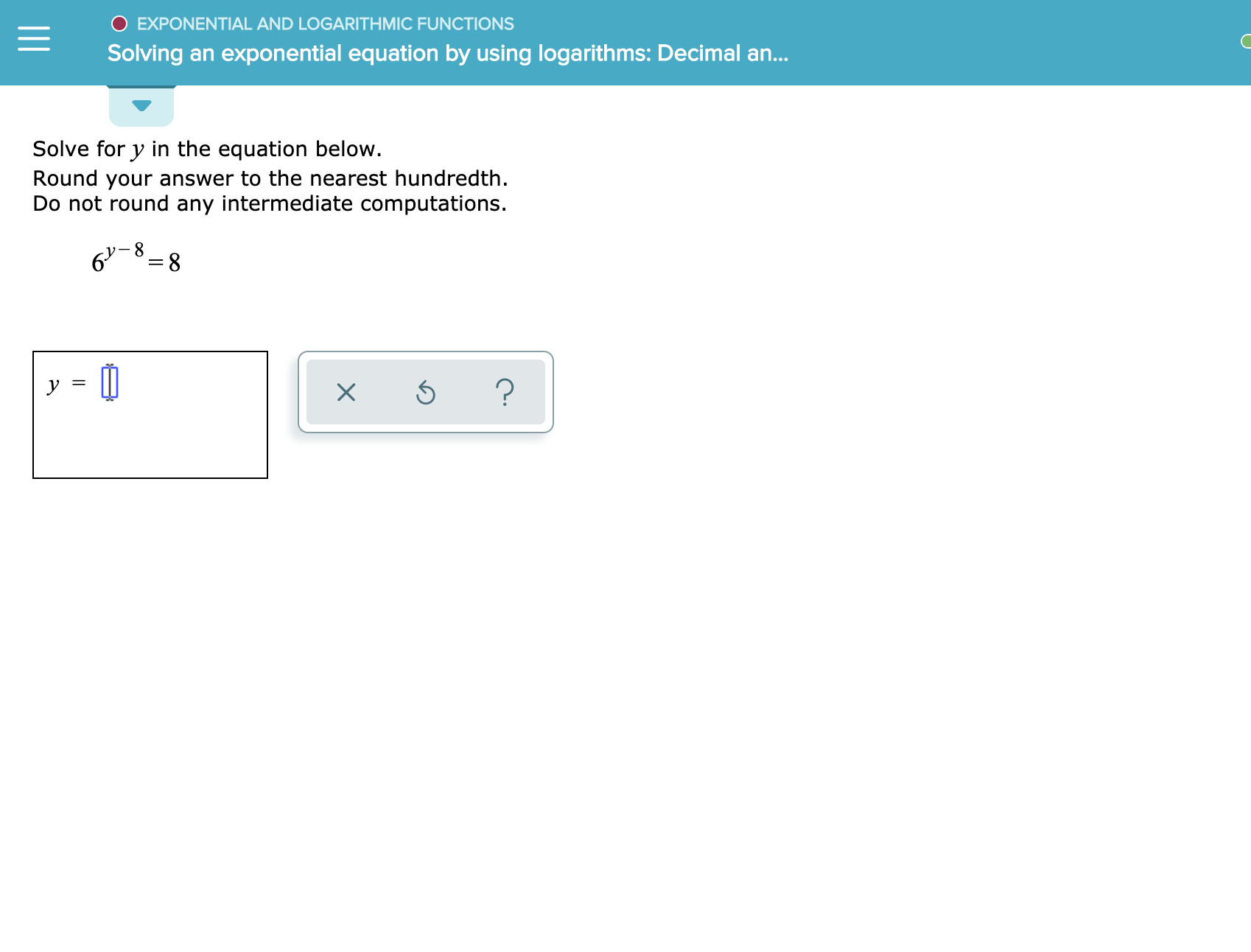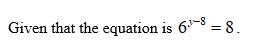# O EXPONENTIAL AND LOGARITHMIC FUNCTIONSSolving an exponential equation by using logarithms: Decimal an...Solve for y in the equation below.Round your answer to the nearest hundredthDo not round any intermediate computations.6 8?X

Question
3 views

see attachedhelp_outlineImage TranscriptioncloseO EXPONENTIAL AND LOGARITHMIC FUNCTIONS Solving an exponential equation by using logarithms: Decimal an... Solve for y in the equation below. Round your answer to the nearest hundredth Do not round any intermediate computations. 6 8 ? X fullscreen
check_circle

Step 1Step 2

Solve the equation for...

### Want to see the full answer?

See Solution

#### Want to see this answer and more?

Solutions are written by subject experts who are available 24/7. Questions are typically answered within 1 hour.*

See Solution
*Response times may vary by subject and question.
Tagged in

### Other